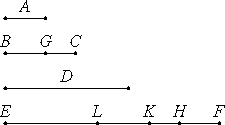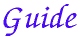# Proposition 36

If as many numbers as we please are in continued proportion, and there is subtracted from the second and the last numbers equal to the first, then the excess of the second is to the first as the excess of the last is to the sum of all those before it.

Let there be as many numbers as we please in continued proportion, A, BC, D, and EF, beginning from A as least, and let there be subtracted from BC and EF the numbers BG and FH, each equal to A.

I say that GC is to A as EH is to the sum of A, BC, and D.

Make FK equal to BC, and FL equal to D.

Then, since FK equals BC, and of these the part FH equals the part BG, therefore the remainder HK equals the remainder GC.And since EF is to D as D is to BC, and as BC is to A, while D equals FL, BC equals FK, and A equals FH, therefore EF is to FL as LF is to FK, and as FK is to FH. Taken separately EL is to LF as LK is to FK, and as KH is to FH.

VII.12

Since one of the antecedents is to one of the consequents as the sum of the antecedents is to the sum of the consequents, therefore KH is to FH as the sum of EL, LK, and KH is to the sum of LF, FK, and HF.

But KH equals CG, FH equals A, and the sum of LF, FK, and HF equals the sum of D, BC, and A, therefore CG is to A as EH is to the sum of D, BC, and A.

Therefore the excess of the second is to the first as the excess of the last is to the sum of those before it.

Therefore, if as many numbers as we please are in continued proportion, and there is subtracted from the second and the last numbers equal to the first, then the excess of the second is to the first as the excess of the last is to the sum of all those before it.

Q.E.D.

###This proposition says if a sequence of numbers a1, a2, a3, ..., an, an+1 is in continued proportion

a1 : a2 = a2 : a3 = ... = an : an+1

then

(a2a1) : a1 = (an+1a1) : (a1 + a2 + ... + an).

This conclusion gives a way of computing the sum of the terms in the continued proportion as

 a1 + a2 + ... + an = a1 an+1 – a1 a2 – a1 .

If we denote the first term by a and the ratio of the terms by r, then this gives the familiar formula

 a + ar + ar2 + ... + arn-1 = a rn – 1 r – 1 .

#### Summary of the proof

The proof is much more comprehisible when it’s translated into algebraic notation. The correspondence is as follows
 A = a1 BC = a2       ... D = an EF = an+1 BG = FH = a1 GC = a2 – a1 EH = an+1 – a1 a2 : a1.

For each proportion, say the first,

an+1 : an = an : an–1,

take it separately according to VII.11 to get

(an+1an) : (anan–1) = an : an–1,

then alternately

(an+1an):an = (anan–1):an–1.

Stringing the conclusions together, we have

(an+1an):an = (anan–1):an–1 = ... = (a2 – a1):a1.

Next, using proposition VII.12, the sum of the antecedents is to the sum of the consequences equals this same ratio. Therefore

(an+1an + anan–1 + ... + a2a1) : (an + an–1 + ... + a2 + a1) = (a2 – a1) : a1.

But an+1an + anan–1 + ... + a2a1 equals an+1 – a1. That gives us the conclusion of the proposition

(an+1a1) : (an + an–1 + ... + a2 + a1) = (a2a1) : a1.

#### Use of this propostion

This proposition is used in the next one.